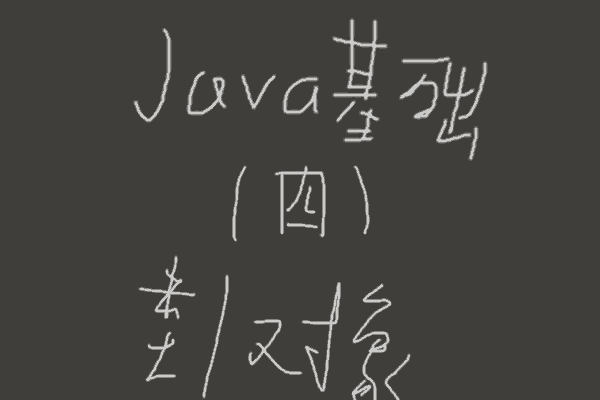# Java基础（四）-类与对象Java基础目录 如果有不好理解的地方，请评论一下，我举例说明。 这部分改了好多次，原本是有两篇的，一篇实用向，一篇个人理解，

#### 代码示例

public class helloTriangle{
public static void main(String args[]){
triangle t = new triangle();  // 定义一个triangle类的t对象
System.out.println("初始三角形周长为：" + t.perimeter()); //输出12
t.a = 5;
System.out.println("t的周长为：" + t.perimeter());  // 输出14
t.changeTriangle(3, 3, 3);
System.out.println("改变t的边长后，周长为：" + t.perimeter());  // 输出9
System.out.println("不使用对象，用类名调用周长函数：" + triangle.perimeter(6, 6, 6));  //输出18

triangle t2 = new triangle(30, 40, 50);
System.out.println("t2周长为：" + t2.perimeter());   // 输出120
}
}

class triangle{  //定义triangle类
// 三角形三边
int a, b, c;

public triangle(){  // 构造方法
a = 3;
b = 4;
c = 5;
}

public triangle(int a, int b, int c){  // 构造方法重载
this.a = a;
this.b = b;
this.c = c;
}

void changeTriangle(int a, int b, int ccc){ // 改变三角形三边值，调用方法时，需传参三个int型数据。
this.a = a;   // 形参a与成员变量a重复，需用this.a表示成员变量a。
this.b = b;
c = ccc;
}

int perimeter(){      // 返回当前三角形周长，上面changeTriangle方法的a b ccc三个参数不影响本方法。
return a+b+c;
}

static int perimeter(int a, int b, int c){  // 重载perimeter方法，且修饰为静态方法，能不通过对象直接调用
return a+b+c;
}
}

#### 类的定义

// 类的结构，[可选项，可不填]
[类修饰符] class 类名称{
[修饰符] 数据类型 成员变量名称 [= 初始值];
...
[修饰符] 返回类型 方法名([参数类型 参数1], [参数类型 参数2], ...){
语句块;
return [表达式];
}
...
}

#### 对象的创建与使用

// 对象的创建与字符串的创建相同，因为字符串对象也是对象。
// 对象的声明

// 对象的创建

// 成员变量赋值

// 带static修饰符的变量可直接用类名赋值

// 成员方法使用

// 带static修饰符的方法可直接用类名调用

#### 类中成员调用类中成员

（因为想名字很难，且Java有机制，所以经常直接用成员变量名作为形参名）

this.方法名效果与之相同。
this关键字用来强调成员属于本类。

#### 方法中的可变参数

[修饰符] 返回类型 方法名(固定参数列表, 可变参数类型 ... 可变参数名){
语句块;
for(可变参数类型 临时变量:可变参数名){  // 使用foreach语句调用可变参数
语句块;
}
}

#### 构造方法

// 构造方法的结构
[修饰符] 类名([参数1], [参数2], ...){
语句块;
}

#### 关于-方法

（可以理解成把地址作为实参传入过去，形参的值就是地址）